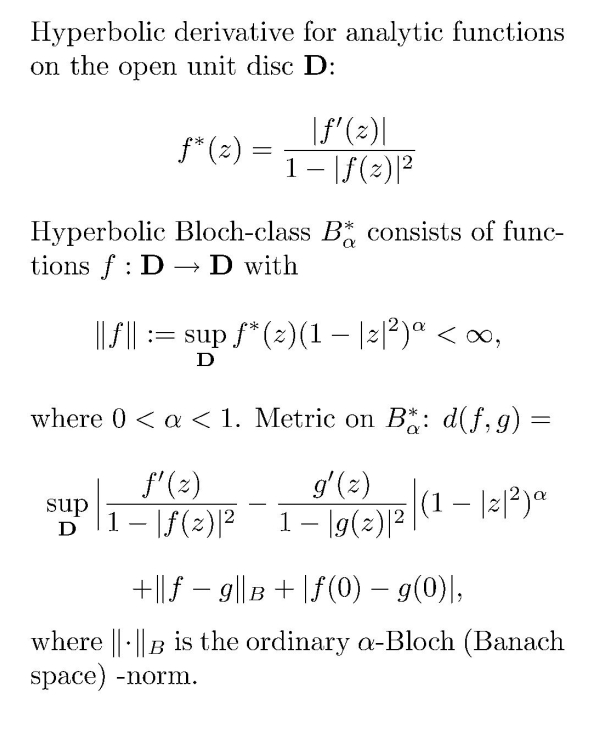Main page Department of Mathematics and Statistics of University of HelsinkiFormula 1. Bloch-to-BMOA-composition operator.Formula 2. Metric on the hyperbolic Bloch class. Let Ω be a complex domain (in one or, respectively, N ≥ 2 dimensions), and let φ: Ω → Ω be an analytic mapping. The analytic composition operator with symbol φ is by definition the mapping f → f o φ, where f belongs to some function space on Ω. SOME RECENT ARTICLES O.Blasco, M.Lindström, J.Taskinen: Bloch-to-BMOA compositions in several complex variables. Complex Var. Theory Appl. 50, 14 (2005), 1061-1080. We study analytic composition operators from the Bloch space to the analytic BMO-space; the domain of the spaces is the unit ball of Cⁿ. Under mild regularity condition for the symbol φ we are able to characterize the boundedness and compactness of the operator in terms of a Carleson measure type condition; see Formula 1. The work is continuation of a paper in Can.Math.Bull. 47,2 (2004). We use a delicate modification of a well known lacunary series argument, which has also been used for example by Choe and Rim in their paper in Acta Math.Hungarica vol. 72. F.Perez-Gonzales,J.Rättyä,J.Taskinen: Lipschiz continuous and compact composition operators on hyperbolic classes. Mediterranean J.Math. 8,1 (2011), 123-135. We study hyperbolic function classes of Bloch, Dirichlet and Qp functions. These are not linear spaces, but we endow them with natural metrics, emerging from their definitions. We prove the completeness of the spaces. The definition of an analytic composition operator still makes sense between these metric spaces. The main result of the paper is that the Lipschitz-continuity plays here the same role as boundedness for usual linear operators in Banach-spaces. We charaterize the Lipschitz-continuity of a composition operator from the hyperbolic Bloch space into hyperbolic Qp in terms of a formula similar to the linear case. We also observe that the natural concept of a compact operator coincides here with the standard definition of completely continuous operator in nonlinear functional analysis. At the end of the paper there is some amusing discussion about the choice of the metrics of the hyperbolic classes, with related, interesting and probably not too difficult open problems. Back to main page .